# Multi Dimensional Arrays in Java

## Multi-Dimensional Arrays in Java with Examples

In this article, I am going to discuss Multi-Dimensional Arrays in Java with Examples. Please read our previous article where we discussed One-dimensional Arrays in Java. At the end of this article, you will understand the following pointers in Java which are related to Java Arrays.

1. What are Multi-Dimensional Arrays in Java?
2. Declaration and Creation of Two Dimensional Array
3. Copying Arrays in Java
4. Passing Arrays to Methods in Java
5. Returning an Array from a Method in Java
##### Multi-Dimensional Arrays in Java:

Multi-Dimensional Arrays means storing multiple values in more than one dimension. The simplest form of Multi-Dimensional Arrays is 2 Dimensional Array.

##### Declaration of Two Dimensional Array

Syntax: datatype arrayname[][];
Example:
int arr[][];
int[][] arr;
int [][]arr;

##### Creation of Two Dimensional Array in Java

Syntax : datatype arrayname[][] = new datatype[size][size];
Example : int arr[][] = new int[][];

##### Rules for Two Dimensional Array
1. When we create a Two Dimensional array specifying the size for the first square bracket is mandatory and for next square bracket, it is optional.
2. Here each square bracket will indicate one single dimensional array.
3. Here multi-dimensional arrays are stored in multiple levels where all top levels of arrays are containing addresses of the next level of arrays and only the last level of arrays contains values.

Example: int A[][] = new int;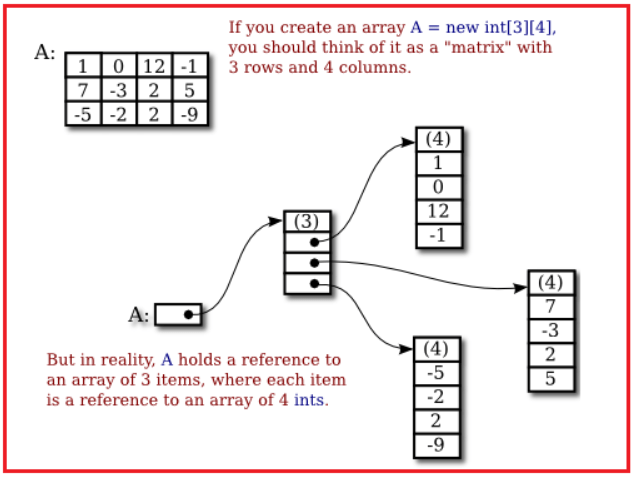Note: In the last level of arrays we may have arrays with an equal number of elements or a non-equal number of elements. We can also write more than 2 dimensions for arrays hence multidimensional arrays are also called an array of arrays.

Example: int arr[][][] = new int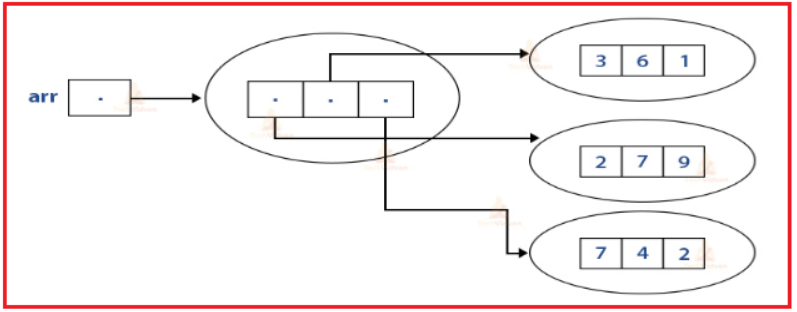###### Sample Program to demonstrate the use of Multi-Dimensional Array in Java
```package Demo;
public class MultidimArrayDemo {
public static void main(String[] args) {
String[][] names = {
{"Mr. ", "Mrs. ", "Ms. "},
{"Smith", "Jones"}
};
// Mr. Smith
System.out.println(names + names);
// Ms. Jones
System.out.println(names + names);
}
}```

Output :##### Copying Arrays in Java

The System class has an arraycopy method that you can use to efficiently copy data from one array into another. The syntax is given below.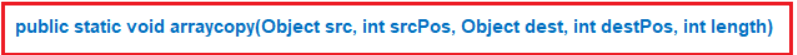The two Object arguments specify the array to copy from and the array to copy to. The three int arguments specify the starting position in the source array, the starting position in the destination array, and the number of array elements to copy.

##### Sample Program to demonstrate the use of arraycopy method in Java
```package Demo;

public class ArrayCopyDemo {
public static void main(String[] args) {
char[] copyFrom = { 'd', 'e', 'c', 'a', 'f', 'f', 'e',
'i', 'n', 'a', 't', 'e', 'd' };
char[] copyTo = new char;

System.arraycopy(copyFrom, 2, copyTo, 0, 7);
System.out.println(new String(copyTo));
}
}```

Output: caffein

##### Passing Arrays to Methods in Java

You can also pass arrays to methods just as you can pass primitive type values to methods.

Example:

public static void printArray(int[] array) {
for (int i = 0; i < array.length; i++) {
System.out.print(array[i] + ” “);
}
}

In the above example, printArray method displays the elements in an int array. You can invoke it by passing an array.

Example: printArray(new int[]{3, 1, 2, 6, 4, 2});

##### Sample Program to pass an array to a method in java
```public class ArrayDemo
{
public static void updateParam (double[]x)
{
x = 9999;	// Update one of the array element
}

public static void main (String[]args)
{
double[] a = { 2.3, 3.4, 4.5 };
System.out.println ("Array before calling updateParam:");

for (int i = 0; i < a.length; i++)
System.out.println (a[i]);

updateParam (a);	// Call updateParam

System.out.println ("Array AFTER calling updateParam:");

for (int i = 0; i < a.length; i++)
System.out.println (a[i]);
}
}```
###### Output: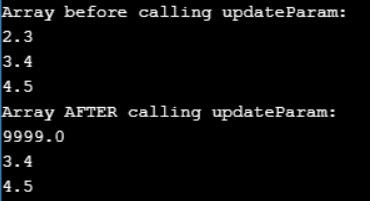##### Returning an Array from a Method in Java

A method may also return an array. An example is given below.

public static int[] reverse(int[] list) {
int[] result = new int[list.length];

for (int i = 0, j = result.length – 1; i < list.length; i++, j–) {
result[j] = list[i];
}
return result;
}

In the above example, the method returns an array that is the reversal of another array.

##### Sample Program to return an array from a method in java:
```import java.util.Arrays;
import java.util.Scanner;
public class ArrayDemo
{
public int[] createArray() {
Scanner sc = new Scanner(System.in);
System.out.println("Enter the size of the array that is to be created:: ");
int size = sc.nextInt();
int[] myArray = new int[size];
System.out.println("Enter the elements of the array ::");

for(int i=0; i<size; i++) {
myArray[i] = sc.nextInt();
}
return myArray;
}

public static void main(String args[]) {
Main obj = new Main();
int arr[] = obj.createArray();
System.out.println("Array created is :: "+Arrays.toString(arr));
}
}```

Output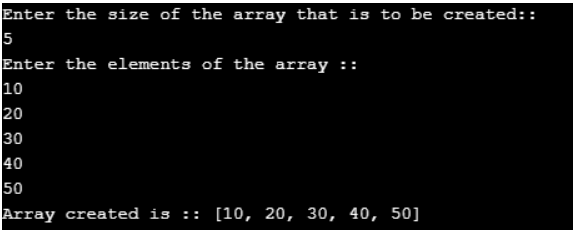In the next article, I am going to discuss the Collections Framework in Java with Examples. Here, in this article, I try to explain Multi-Dimensional Arrays in Java with examples. I hope you enjoy this Multi-Dimensional Arrays in Java with Examples article. I would like to have your feedback. Please post your feedback, question, or comments about this Multi-Dimensional Arrays in Java with Examples article.